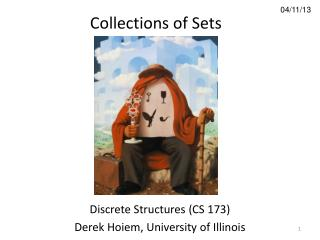DownloadDownload Presentation04/11/13

# 04/11/13

Download Presentation## 04/11/13

- - - - - - - - - - - - - - - - - - - - - - - - - - - E N D - - - - - - - - - - - - - - - - - - - - - - - - - - -
##### Presentation Transcript

1. Collections of Sets 04/11/13 Discrete Structures (CS 173) Derek Hoiem, University of Illinois

2. Today’s class • Collections of sets: basics • Review of midterm

3. Midterm grade distribution min 23 median 81 mean 78 max 100 pctl grade 10 56 20 68 30 73 40 78 50 81 60 85 70 88 80 91 90 94

4. Example: Image segmentation • An image is divided into regions based on color or other appearance features • Each region is a set of pixels • The segmentation is a collection of regions, or a collection of sets of pixels

5. Sets of sets

6. The powerset of set is the set of all possible subsets of Example: the powerset of is Example: the powerset of the set of pixels is the set of all possible image regions (including discontinuous regions) If we have pixels, how many possible regions are there?

7. Functions on sets Powersets most often used for defining the domain or co-domain of a function that operates on sets Example 1: function to compute the mean intensity of pixels in a region is a set of pixel indices is an element of (an image index) is the pixel intensity

8. Functions on sets Powersets most often used for defining the domain or co-domain of a function that operates on sets Example 2: function to return indices of red pixels is an element of (an image index) is the intensity in red channel

9. Partitions There are 10 kinds of people: those who understand binary and those who don’t. A partition of is a collection of non-empty subsets of , such that each element of is contained by exactly one subset

10. Partitions with Finite Sets A collection of sets is a partition of • : • No overlap within elements of : if • No element of is empty The segmentation is a partition of the image pixels: the regions do not overlap, are non-empty, and their union includes all pixels

11. Partitions with Infinite Sets A collection of sets is a partition of if Example 1: The set of natural numbers can be partitioned into those between 0 and 9, 10 and 99, 100 and 999, and -1, etc. (elements of each set have the same number of digits) Example 2: Any elements of the set of real numbers that round to the same integer are in a set. The collection of these sets is a partition on the set of real numbers.

12. Partitions of continuous spaces Quantization: map to a partition of • Clustering • Decision trees Represent any points that fall into each cell with an integer Treat all points within a cell as the same Represent points in cell with summary statistics, such as mean Simplifies matching and function fitting

13. Partition of continuous space (R2) Population density by state Population density by county Population distribution over area approximated by population within each state or county Source: http://en.wikipedia.org/wiki/List_of_U.S._states_by_population_density

14. Things that are not partitions is not a partition of is not a partition of , is not a partition of the natural numbers , is not a partition of the natural numbers

15. Some nit-picky things to remember • Important to distinguish between a one-element set and an element: • Common programming error (e.g., function is expecting a set but gets a number) • Every powerset contains the empty set as an element • An element of a collection (or set of sets) is a set

16. Review of midterm

17. Next week • Combinations and counting • Dice rolls and poker hands • Finite state machines